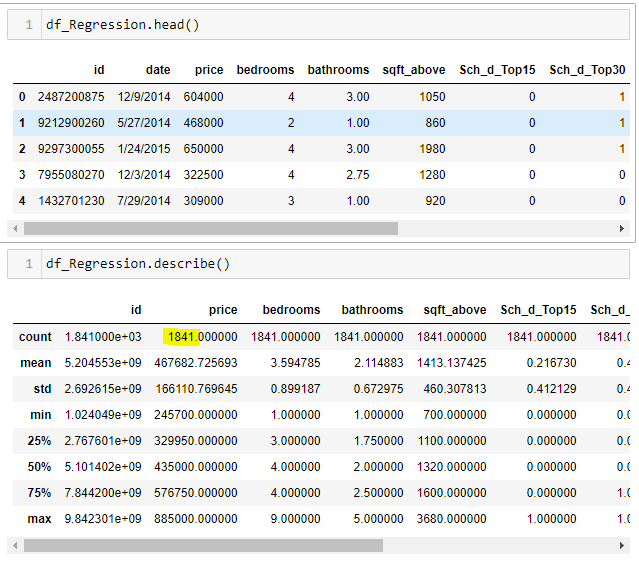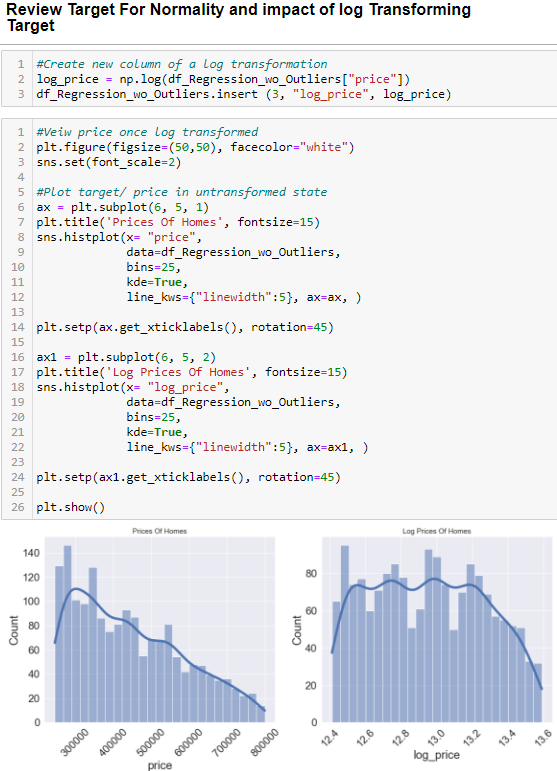# Using the Past to Predict the Future

Part 1 of 3, Creating a Regression Model in Python

# Introduction

Using the past to predict the future! Say hello to Regression Modeling! In this three-part series I will show you how to create, use, and check the validity of a regression model with python. To effectively cover the topic, I have broken the topic into the following parts.

Blog 1 (this blog)

1. Overview of Data/ Understand the Business Objectives
2. Processing your Data
a. Load/ Study/ Cleanse Data
b. Review Data Types, Convert Categorical to Dummies (Removed here for sake of brevity)
c. Check For & Remove Extreme Outliers
d. Ensure Linearity and Check/ Remove Multicollinearity
e. Review/ Transform Distribution of Target Variable
f. Transform/ Scale Feature Data (if Required)
g. Split Data (Removed here for sake of brevity)

Blog 2

3. Create/ Run Model/ Reuse

Blog 3

4. Checking Remaining Linearity Assumptions — Homoscedasticity & Normality in residuals

For a more thorough overview of the project related to this series of blogs see: https://github.com/rgpihlstrom/Phase2Project

Blog assumptions: Given the need for brevity I will assume the reader has some basic knowledge of regression and how a regression model is structured. However, I will try to cover the basic foundational principles with the assumption that this blog series is targeted toward the beginner. :)

Now lets get started!

# 1. Overview of Data/ Understanding Business Objectives

The Data: We are using a combination of King County Housing Data along with some ancillary data scraped from the King County Tax Assessor Records as well as School District Ranking data from King County Gov. The King County Housing Data captures the actual sales of homes that took place between 5/14-5/15 in King County Washington.

Business Objective: Help a local real estate firm use past sales data to predict potential future prices on the sale of homes. Furthermore, help sellers understand what features are most impactful on improving the value of their homes.

• Target/ Dependent Variable: price
• Features/ Independent Variables: bedrooms, bathrooms, sqft_above,Sch_d_Top15, Sch_d_Top30, Sch_d_Top60, AppraisedLandValue, AppraisedHomeValue
• Meta Data: id, date. Id = Parcel ID, Date = Date of Sale

# 2. Processing Our Data — The Fun Starts Now!

GARBAGE IN GARBAGE OUT! As the adage goes, so goes your model. Using linear regression requires adhering to the following assumptions:

## Pre-Model Assumptions - Covered Here

1. Linearity between Target and Features

2. No Multicollinearity between Features

## Post Model Assumptions - Covered in Blog 3

3. Homoscedasticity in Residual Error

4. Normality in the Residual Error

`# Import appropriate librariesimport pandas as pdimport numpy as np#Importing data into dataframe.  This data is available from 3 sources,  Using Parcel ID you can link this data across sources 1. Kaggle @ https://www.kaggle.com/harlfoxem/housesalesprediction 2.https://blue.kingcounty.com/Assessor/eRealProperty/Dashboard.aspx3. https://backgroundchecks.org/top-school-districts-in-washington-2018.htmldf_Regression = pd.read_excel("df_Regression_Blog.xlsx")`Preview of data, we have 11 columns and 1841 rows

Find If Your Data Contains Any Nulls

`#Check for missing valuesmissing = df_Regression.isnull().sum()if (missing > 1).any():   missing = df_Regression[missing > 0]   print (missing)else:   print("Thankfully no missing values! YAY!")`

B. Review Data Types, Convert Categorical to Dummies –Categorical features are often present in your data. For this blog, columns “Sch_d_Top15, Sch_d_Top30, Sch_d_Top60, Sch_d_Top100” are categorical features. These columns were the output of previously performed processing using one-hot encoding. For more information on categorical data and one-hot encoding see here. For the sake of brevity I have excluded going into depth here.

C. Check For & Remove Extreme Outliers. Why are outliers important and why should we remove them from our dataset? Your model is “trained” by the data. What that means is your model will be optimized to infer/ predict values from the features that are most common/ frequent in your data. Outliers will “pull”/ influence your model as it tries to account for those values. A key point to note here is that one model may not account for all cases. If you have a lot of data, it is possible that you may need more than one model to explain all variability accurately. This is the case in our situation. The model presented in this blog was optimized for the “most common” values in our features. By doing this we create a more accurate model for the homes that are most prevalent in our data.

`# Continuous features/ columnsdf_Features_To_Check = df_Regression[['price','bedrooms', 'sqft_above','AppraisedLandValue', 'AppraisedHomeValue']]# Set styles on plots when showing boxplotplt.figure(figsize=(60,40), facecolor="white")plt.subplots_adjust(hspace = 0.8)sns.set_style('whitegrid')sns.set(font_scale=5)# Loop through continuous features and show boxplotfor index, column in enumerate(Features_To_Review):ax = plt.subplot(4, 3, index+1)sns.boxplot(x=column, data=df_Features_To_Check, ax=ax);plt.show();`

Notice the highlights, some data point should be considered outliers. But how much data should we remove? This question is up to you. You may have to revisit how much data you keep and or remove from your model based on the results of your model which will not be available until further steps in the process. Generally speaking, we would like approximately 1000 rows of data to use in our final model. Below are a few lines of code to help us understand the number of rows being removed by filtering our dataframe for shown highlighted violators.

`# Set "Cut Off" Valuesprice_cut_off = 800000bedrooms_upper_cut_off = 5bedrooms_lower_cut_off = 2sqft_above_cut_off = 3000AppraisedLandValue_cut_off = 400000AppraisedHomeValue_cut_off = 550000# Get a sense of the number of rows potentially being cut off with by removing outliers#Prices_price = df_Regression.apply(lambda x: True if x['price'] > price_cut_off else False , axis=1)numOfRowsCutting_price = len(s_price[s_price == True].index)print(f' Price Rows Cutting = {numOfRowsCutting_price}')#Bedroomss_bedrooms = df_Regression.apply(lambda x: True if ((x['bedrooms'] > bedrooms_upper_cut_off) or(x['bedrooms'] < bedrooms_lower_cut_off)) else False , axis=1)numOfRowsCutting_bedrooms= len(s_bedrooms[s_bedrooms == True].index)print(f' Bedrooms Rows Cutting = {numOfRowsCutting_bedrooms}')#Squarfoots_sqft_above = df_Regression.apply(lambda x: True if x['sqft_above'] > sqft_above_cut_off else False , axis=1)numOfRowsCutting_sqft_above = len(s_sqft_above[s_sqft_above == True].index)print(f' sqft_above Cutting = {numOfRowsCutting_sqft_above}')#AppraisalValuess_AppraisedLandValue = df_Regression.apply(lambda x: True if x['AppraisedLandValue'] > AppraisedLandValue_cut_off else False , axis=1)numOfRowsCutting_AppraisedLandValue = len(s_AppraisedLandValue[s_AppraisedLandValue == True].index)print(f' AppraisedLandValue Rows Cutting {numOfRowsCutting_AppraisedLandValue}')s_AppraisedHomeValue = df_Regression.apply(lambda x: True if x['AppraisedHomeValue'] > AppraisedHomeValue_cut_off else False , axis=1)numOfRowsCutting_AppraisedLandValue = len(s_AppraisedHomeValue[s_AppraisedHomeValue == True].index)print(f' AppraisedHomeValue Rows Cutting = {numOfRowsCutting_AppraisedLandValue}')`

Based on the above I will remove the rows using the shown criteria

`#Remove outliers rows using criteria abovedf_Regression_wo_Outliers = df_Regression[(df_Regression["price"] < price_cut_off)& (df_Regression['bedrooms'] <= bedrooms_upper_cut_off)& (df_Regression['bedrooms'] >= bedrooms_lower_cut_off)& (df_Regression["sqft_above"] <= sqft_above_cut_off)& (df_Regression["AppraisedLandValue"] <= AppraisedLandValue_cut_off)& (df_Regression["AppraisedHomeValue"] <= AppraisedHomeValue_cut_off)]`

We removed approximately 200 outliers

D. Ensure Linearity & Check/ Remove Multicollinearity - (pre-model assumptions).

Linearity — We check for linearity via a scatter plot(s), plotting each feature against our target. Below are scatter plots for each continuous feature

`# Plot our target against our featuresplt.figure(figsize=(60,40), facecolor="white")sns.set(font_scale=2)for index, column in enumerate(df_Features_To_Check):   if column != "price":   ax = plt.subplot(6, 5, index+1)   sns.regplot(x=column, y="price", data=df_Features_To_Check,   ax=ax, label='medium', line_kws={"color": "red"});   plt.ticklabel_format(style='plain', axis='both')   plt.xticks(fontsize=30)   plt.yticks(fontsize=30)plt.show();`

Based on the above, all features are linearly related to the target. As our features increase our target also increases. Therefore, we are okay to proceed in checking for the next assumption.

Multicollinearity —We check for multicollinearity using Variance Influence Factor (VIF). Looking for scores < 10. For more information on multicollinearity see here. Checking for multicollinearity is necessary as the linear regression modeling is predicated on each variable impacting our target variable independently from each other. If multicollinearity is present in our data it means that as one feature changes then another feature is changing with it systematically. This violates the principle of linear regression.

`#import VIFfrom statsmodels.stats.outliers_influence import variance_inflation_factor#Feed continuous columns into VIFdf_ColsToCheck = df_Regression_wo_Outliers[["sqft_above","bedrooms","AppraisedLandValue", "AppraisedHomeValue"]]vif = [variance_inflation_factor(df_ColsToCheck.values, i) for i in range(df_ColsToCheck.shape)]vifresults = list(zip(df_ColsToCheck.columns, vif))vifresults`

As you can see, we have 3 values that are higher than 10. To correct for this there are a few options. For this blog I am going to drop bedrooms and combine our Appraisal values columns to create a combined total called “TotalAppraisalValue”. Then I will retest to ensure I have corrected our VIF scores.

`df_Regression_wo_Outliers["TotalAppraisalValue"] = df_Regression_wo_Outliers["AppraisedLandValue"] + df_Regression_wo_Outliers["AppraisedHomeValue"]#Drop columns that did not pass VIF Testdf_Regression_wo_Outliers = df_Regression_wo_Outliers.drop(columns=['bedrooms','bathrooms','AppraisedLandValue','AppraisedHomeValue'])`

Based on our new results we have satisfied this assumption and can continue with additional pre-model processing.

E. Review/ Transform Distribution of Target Variable

What does this step mean, and why is it important? A “Normally” distributed target will help create a more robust model. To check the normality of our target variable we generate a plot showing our target in the current untransformed state as well as a log transformed state. If transforming helps make a more normalized distribution then we opt for the transformed state. The drawback is interpretability. However, we can reverse this transformation further in our process. More on this topic in Blog 2. For more information on why you transform variables see here.

`#Create new column of a log transformationlog_price = np.log(df_Regression_wo_Outliers["price"])df_Regression_wo_Outliers.insert (3, "log_price", log_price)#Veiw price once log transformedplt.figure(figsize=(50,50), facecolor="white")sns.set(font_scale=2)#Plot target/ price in untransformed stateax = plt.subplot(6, 5, 1)plt.title('Prices Of Homes', fontsize=15)sns.histplot(x= "price",data=df_Regression_wo_Outliers,bins=25,kde=True, line_kws={"linewidth":5}, ax=ax, )plt.setp(ax.get_xticklabels(), rotation=45)ax1 = plt.subplot(6, 5, 2)plt.title('Log Prices Of Homes', fontsize=15)sns.histplot(x= "log_price1",data=df_Regression_wo_Outliers,bins=25,kde=True,line_kws={"linewidth":5}, ax=ax1, )plt.setp(ax1.get_xticklabels(), rotation=45)plt.show()`Comparing Normal vs. Log Transformed Distribution

As you can see in the above, our transformed target, price, is closer to a normal distribution (graph on right) than an untransformed price. Given the benefits associated with a more normally distributed target on the models ability to predict we will log transform price. At this point you can drop price from your model; I prefer to keep this column for future reference as it aids in interpretability.

F. Scaling Features
Similar to transforming our target variable (shown above), feature scaling and even transformation is fairly standard in regression model creation. See here for more information on why and when feature scaling is needed. Below I will use MinMax Scaling. MinMax helps us determine which feature has the most impact on the model. We will see the result of this in blog 2.

`# Import library required to scale datafrom sklearn.preprocessing import MinMaxScaler# Create dataframe to feed into scaler, make sure not to feed scaler your categorical datadf_Data_To_Be_Scaled = df_Regression_wo_Outliers[["sqft_above","TotalAppraisalValue"]]# Initiate Scalerscaler = MinMaxScaler()# Create instance of scaler, this step is required for future usage beyond initial dataset/ model creationscaler = MinMaxScaler().fit(df_Data_To_Be_Scaled)# Transform data, output arrayscaled_Data = scaler.fit_transform(df_Data_To_Be_Scaled)# Create dataframe of scaled valuesdf_scaled_Data = pd.DataFrame(scaled_Data, columns=[["sqft_above_sc","TotalAppraisalValue_sc"]])df_scaled_Data = df_scaled_Data.reset_index(drop=True)`

F. Split Test, Train Data

Normally the last step you perform prior to creating your model is to split your data into two parts, one part to train/ create your model and another part to test your model. For the sake of brevity, I have forgone showing this step here as it will not impact my coverage of the remaining steps but will certainly add to the length of this blog 😊. For more information on train, test splitting see here.

# Conclusion & Preview of Blogs 2 & 3

Above I covered the steps required to get your data ready prior to model creation. It starts with having a firm grasp of the business objective you are trying to achieve with your regression model. Next, I outlined a few techniques in identifying nulls, finding and removing outliers which will negatively impact your model. Next, I demonstrated how to check for the first two, pre-model, assumptions associated with creating a valid linear regression model. Lastly, I showed you how to transform and scale your target and feature variables. In blog post 2 I will build on the above, showing you how to create the actual model as well as use the model after initial creation. In blog 3 I will review how to check for the last two assumptions associated with a linear regression modeling. I look forward to seeing you again and finishing the introduction to regression modeling in the aforementioned future posts.

Next Stop — DATA ALCHEMY!

--

--